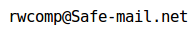## GlobeCalc

GlobeCalc ("Global Calculator") is a C language library of computational primitives that promotes "Round World Computing", by performing fundamental engineering calculations with the mathematical representation of the planetary surface and locations on it.

GlobeCalc Library computations assume that the planetary surface is a spheroid (an ellipsoid of rotation, represented by gcPlanet data object, see below), and that an atomic location descriptor on this surface (gcPoint, see below) is an opaque data object, that describes the location on the planetary surface with some finite, millimetric resolution. All the computations are therefore performed with a finite precision, commensurate with the gcPoint granularity. The library also provides a set of functions that return the differences between a selected set of such computations and their equivalent ellipsoidal variants.

The fundamental data types of the GlobeCalc library are:

gcPlanet is a data object consisting of several double precision numbers, initialised by gcInitPlanet() function. It represents the spheroidal planetary surface as a mathematical abstraction: an ellipsoid of rotation.

gcLatLong is a data object consisting of two double precision numbers, radian measurements of latitude and longitude of a point on the planetary surface represented by an associated gcPlanet object. gcLatLong is an application-semantic rooted numerical representation of a point on Earth (or some other spheroidal planetary surface).

gcPoint is an eight-byte integer that represents an atomic location (point, vertex, "0-cell"...) on the surface of spheroidal body, in a compact form well suited for storage in the computer systems, as well as spherical and spheroidal calculations. On the planet the size of Earth, the precision of gcPoint is about 6 millimetres (mean, 20 millimeters maximum). gcPoint is transformed from and into gcLatLong object by gcPointToLatLong() and gcLatLongToPoint() functions.

gcDelta is an opaque two byte object that represents the position "rounding error", incurred by transforming a location expressed by a gcLatLong object into a corresponding gcPoint object. If an application requests this value while calling gcLatLongToPoint() and provides it to gcPointToLatLong(), the location returned by the latter is guaranteed to differ less than a third of a millimeter from the one supplied to the former.

gcProximity is an opaque double precision number that describes the proximity relationship between two gcPoint objects. gcProximity is not the same as the distance between two objects, but it has one important property relative to it: in any given vicinity, "proximities" (implied by the gcProximity number magnitude) will order the same as the distances. gcProximity may be transformed into geodesic distance with more or less precision, depending on the items (in addition to the gcPlanet) known and the precision required.

As of this writing (2011-05-29), GlobeCalc is work in progress. A brief inventory of library functions can be consulted [here]. "Round World" computation geometry fundamentals are depicted [here].

### Distribution and licensing

GlobeCalc Library is distributed in source form and can be compiled on many (if not most) 32 and 64 bit platforms. GlobeCalc source code is protected by copyright and distributed under "BSD-style" [license]: this means that the Library can be used without payment by both commercial and non-commercial software applications, whether they are "open" or "closed" source.

If you require further information or if you would like to use the Library in your software project, write to Round World Computing at:...